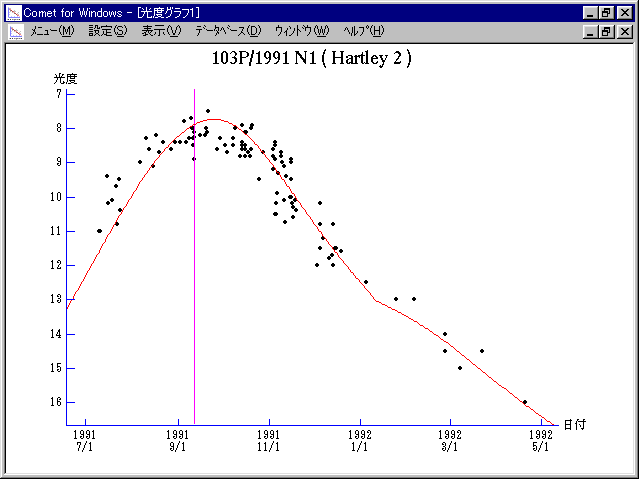# 103P/Hartley 2 (1991)###Profile

 Designation 103P/1991 N1 Recovery Date July 9, 1991 Magnitude 11 mag Recoverer T. V. Kryachko (Majdanak)

###Orbital Elements

```                    Epoch = 1991 Sept.21.0 ET
T = 1991 Sept.11.6542 ET         Peri. = 174.9337
e = 0.719489                     Node  = 226.0539  1950.0
q = 0.953285 AU                  Incl. =   9.2561
a =  3.398382 AU    n = 0.1573243    P =   6.265 years
```

###Magnitudes Graph

```        m1 =  8.2 + 5 log d + 20.0 log r(t - 20)  [   ,120]  (             - 1992 Jan.  9)
m1 = 10.0 + 5 log d + 10.0 log r          [120,   ]  (1992 Jan.  9 -             )
```##### The orbital elements are calculated by Dr. Green and printed on IAUC 5324. The magnitudes graphs are made with Comet for Windows.